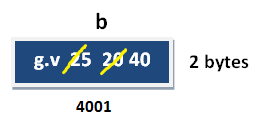# Variable in C++

## Variable in C++

Variable is an identifier which holds data or another one variable is an identifier whose value can be changed at the execution time of program. Variable is an identifier which can be used to identify input data in a program.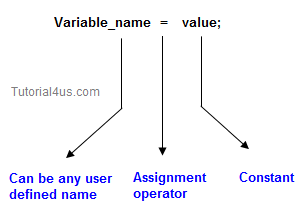## Syntax

```Variable_name = value;
```

## Variable declarations

This is the process of allocating sufficient memory space for the data in term of variable.

## Syntax

Datatype variable_name; int a;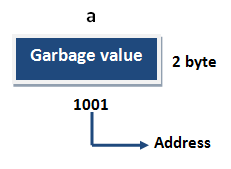If no input values are assigned by the user than system will gives a default value called garbage value.

## Garbage value

Garbage value can be any value given by system and that is no way related to correct programs.
It is a disadvantage and it can overcome using variable initialization.

## Variable initialization

It is the process of allocating sufficient memory space with user defined values.

## Syntax

```Datatype nariable_name=value;
```

## Example

```int  b = 30;
```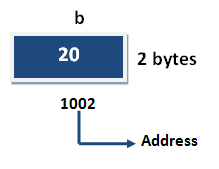## Variable assignment

It is a process of assigning a value to a variable.

## Syntax

Variable_Name = value

## Example

```int  a= 20;
int b;
```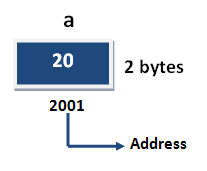## Example

```b = 25; // --> direct assigned variable
b = a;  // --> assigned value in term of variable
b = a+15;  // --> assigned value as term of expression
```